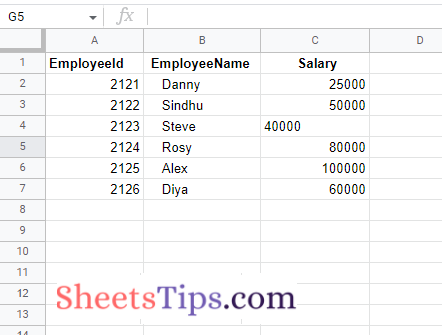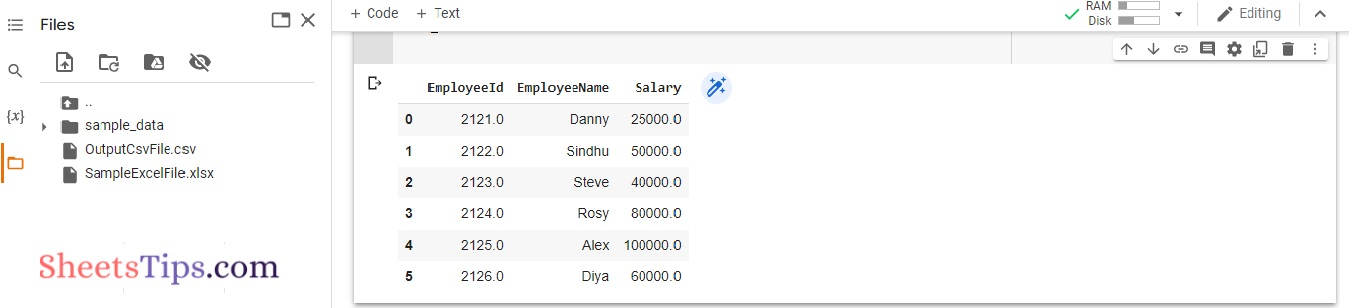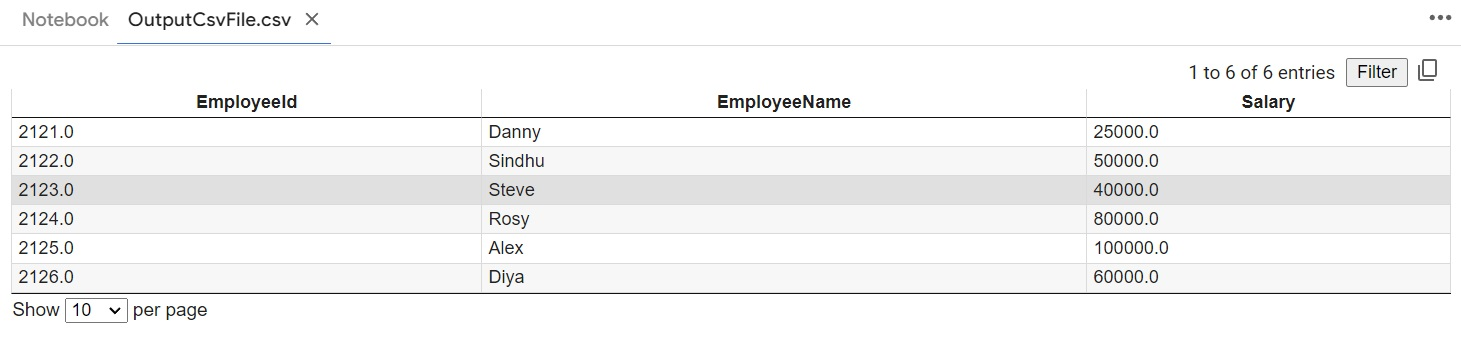# How to Convert Excel to CSV in Python? – Conversion of Multiple CSVs to Excels

CSV is one of the most widely used file formats across the globe for viewing data in a tabular format. Though we can view the data in the tabular format in Excel, there are a few disadvantages, such as slow Excel sheets that consume large amounts of storage space and much more. To overcome this issue, we can simply opt for the CSV file format since it consumes less space and is also very fast. Thus, it is always better to convert the Excel files into CSV and share or store them without any difficulty. There are many ways with the help of which we can convert Excel files to CSV. However, converting the Excel files to CSV using Python is much easier, which is discussed on this page.

On this page, we have explained how simple it is for users to convert the Excel files to the CSV file format. Scroll down to find out more.

## How Do I Convert a CSV File to Excel Without Opening It?

This post will cover the conversion of an Excel (.xlsx) file to a .csv file. Excel mostly includes two formats:

• Excel Microsoft Office Open XML Format Spreadsheet file – (*.xlsx).
• Excel Spreadsheet(Excel 97-2003 workbook) –  (*.xls)

Here we will use the below Excel spreadsheet as an example and convert it into a CSV File.

SampleExcelFile:We can Convert the Excel File into a CSV File in multiple ways. The following ways can be used to Convert the Excel File into a CSV File:
• Using Pandas Library.
• Using xlrd and CSV library.
• Using openpyxl and CSV library.

## Python Program to Convert Excel File to CSV File

### Method #1: Using Pandas Library

Pandas is an open-source data manipulation and analysis library in Python.  It provides a wide range of data structures and operations for manipulating numerical tables and time series. It can read, filter, and re-arrange small and large datasets and output them in Excel, JSON, and CSV formats.

To read an excel file, use the read_excel() function, then to convert the data frame to a CSV file, use the pandas to_csv() function.

Approach:

• Import pandas module using the import keyword
• Read some random excel file using the read_excel() function of the pandas module by passing the file name/path as an argument to it and store it in a variable.
• Apply to_csv() function on the above excel file by passing some random file name index as None, header= True as arguments to write the dataframe object into CSV file.
• Read the above-obtained output CSV file using read_csv() file function and convert into a dataframe object using the DataFrame() function of the pandas module.
• Store it in a variable.
• Display the above dataframe object.
• The Exit of the Program.

Below is the implementation:

```# Import pandas module using the import keyword
import pandas as pd

# Read some random excel file using the read_excel() function of the pandas module
# by passing the file name/path as an argument to it and store it in a variable.

# Apply to_csv() function on the above excel file by passing some random file name
# index as None, header= True as arguments to write the dataframe object
# into csv file
gvn_ExcelFile.to_csv ("OutputCsvFile.csv", index = None, header=True)

# Read the above obtained output csv file using read_csv() file function and convert
# into a dataframe object using the DataFrame() function of the pandas module.
# Store it in a variable.

# Display the above dataframe object
rslt_dataframe
```

Output:### Method #2: Using xlrd and CSV library

xlrd is a library that is used to read Excel files.

csv is a library that allows you to read and write CSV file.

Approach:

• Import xlrd module using the import keyword.
• Import CSV module using the import keyword.
• Import pandas module using the import keyword.
• Pass the excel file path to the open_workbook() function of the xlrd module and access the first sheet using sheet_by_index().
• Store it in a variable.
• Create a CSV file using the writer() function and store it in a variable.
• Loop through each row of the worksheet and write the data into output_CSV_file.
• Write the data into the output csv file row-wise using the writerow() function.
• The Exit of the Program.

Below is the implementation:

```
# Import xlrd module using the import keyword
import xlrd
# Import csv module using the import keyword
import csv
# Import pandas module using the import keyword
import pandas as pd

# Pass the excel file path to the open_workbook() function of xlrd
# module and access the first sheet using sheet_by_index().
# Store it in a variable
worksheet = xlrd.open_workbook("SampleExcelFile.xlsx").sheet_by_index(0)

# Create a csv file using the writer() function and store it in a variable.
OutputCsvFile = csv.writer(open("OutputCsvFile.csv", 'w'), delimiter=",")

# Loop thought each row of the worshsheet and write the data into output csv file
for eachrow in range(worksheet.nrows):
# Write the data into the output csv file row-wise using the writerow() function

OutputCsvFile.writerow(worksheet.row_values(eachrow))```

Output:### Method #3: Using openpyxl and CSV library

openpyxl is a Python module for reading and writing Excel 2010 xlsx/xlsm/xltx/xltm files. It arose from the lack of an existing library that allowed Python to read and write the Office Open XML format natively.

What is a CSV File?

The most common import and export format for spreadsheets and databases is CSV (Comma Separated Values). Because there is no “CSV standard,” the format is defined operationally by the numerous apps that read and write it. Because there is no standard, there are typically small variances in the data produced and consumed by different applications. These distinctions can make processing CSV files from several sources inconvenient. Even though the delimiters and quotation characters differ, the general structure is similar enough that a single module can quickly process such data while obscuring the details of reading and writing the data from the programmer.

csv module in Python:

The csv module includes classes for reading and writing CSV tabular data. It enables programmers to say things like “put this data in the format preferred by Excel” or “read data from this file generated by Excel” without having to know the specifics of the CSV format used by Excel. Programmers can also describe the CSV formats that other apps understand or create their own special-purpose CSV formats.

Approach:

• Import openpyxl module using the import keyword
• Import csv module using the import keyword
• Import pandas module using the import keyword
• Pass the excel file path to the load_workbook() function of openpyxl module to load the excel file and store it in a variable
• Apply active attribute on the given excel file to select the active sheet and store it in another variable
• Create a csv file using the writer() function and store it in a variable.
• Loop through each row of the worshsheet using the for loop and write the data into output csv file
• Write the data into the output csv file row-wise using the writerow() function.
• The Exit of the Program.

Below is the implementation:

```# Import openpyxl module using the import keyword
import openpyxl
# Import csv module using the import keyword
import csv
# Import pandas module using the import keyword
import pandas as pd

# Pass the excel file path to the load_workbook() function of openpyxl
# module to load the excel file and store it in a variable

# Apply active attribute on the given excel file to select the active sheet
# and store it in another variable
worksheet = gvn_ExcelFile.active

# Create a csv file using the writer() function and store it in a variable.
OutputCsvFile = csv.writer(open("OutputCsvFile.csv", 'w'), delimiter=",")

# Loop through each row of the worshsheet using the for loop and write the data
# into output csv file
for eachrow in worksheet.rows:
# Write the data into the output csv file row-wise using the writerow() function
OutputCsvFile.writerow([cell.value for cell in eachrow])
```

Output:Converting XSLV to CSV using Python Pandas is much easier if you simply follow the steps listed above. So, to avoid storage issues, follow the method provided on this page and convert your Excel files to CSV once you are done with the work.